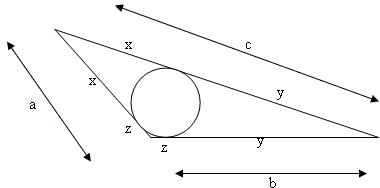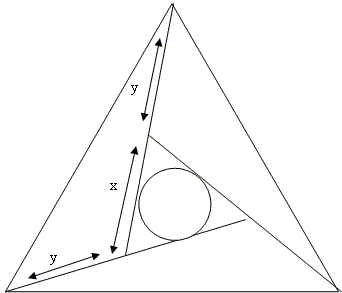# Four Incircles in Equilateral Triangle: What Is This About? A Mathematical Droodle

### This applet requires Sun's Java VM 2 which your browser may perceive as a popup. Which it is not. If you want to see the applet work, visit Sun's website at https://www.java.com/en/download/index.jsp, download and install Java VM and enjoy the applet.

 What if applet does not run?

Explanation# Four Incircles in Equilateral Triangle

Here is a sangaku - a Temple geometry - problem in an equilateral triangle similar to another one in a square. The similarity aside, the current one is much more difficult than the other.

This is the problem #2.1.7 from [Fukagawa and Pedoe]. The problem is from an existent 1886 tablet found in the Fukusima prefecture:

In an equilateral triangle ABC the lines AC'A', BA'B', CB'C' are drawn making equal angles with AB, BC, CA, respectively, forming the triangle A'B'C', and so that the radius of the incircle of triangle A'B'C' is equal to the radius of the incircle of triangle AA'B. Find A'B' in terms of AB.

### This applet requires Sun's Java VM 2 which your browser may perceive as a popup. Which it is not. If you want to see the applet work, visit Sun's website at https://www.java.com/en/download/index.jsp, download and install Java VM and enjoy the applet.

 What if applet does not run?

The answer to the problem is

A'B' = AB·3 / (3 + 21).

Unhappily I must confess that the equations I am able to write appear unappealing. I just could not see any shortcuts that would lead to an elegant solution.

Having no solution, I do not know whether the following observation is relevant or not: lines AA', BB', CC' touch, by the construction three of the four incircles. When the four have equal radii, the lines parallel to the former but passing on the other side of the incircle of ΔA'B'C' seem to also touch three of the four circles.

A later addition: George Zettler kindly sent me the following solution (6/16/2008).

First up, let's establish some general rules about triangles containing one angle of 120° (let's call them 120-triangles). We will denote the sides of such a thing as {a, b, c} where "c" will denote the side opposite the 120° angle.

Claim 1: the radius of the incircle in a 120-triangle is 3(a + b - c)/2.

Proof: This goes much like the analogous calculation for a right triangle. We have the picture:We remark that r, the radius in question, is 3z (this is an easy calculation, using the 120° angle, and the kite which contains it). But it is obvious that c = a + b - 2z and the formula follows at once.

Claim 2: c² = a² + b² + ab.

Proof: this is a variant of the Pythagorean Theorem (or, if you know trig, it is just a special case of the Law of Cosines). From the picture we have:The right triangle on the left is clearly a 30-60-90 triangle, from which it follows that q = a/2, and that p = 3a/2. The claim follows at once from the Pythagorean Theorem applied to the big right triangle.

Now, we need to look at the temple problem. The 3 congruent triangles are clearly 120-triangles. The inner one is equilateral. It is easy to work out the radius of the incircle in an equilateral triangle of side length x, it is x/(23).

The rest is algebra! Granted, it isn't terribly pretty algebra. We divide the sides of the 120-triangles into x and y, with x the side of the equilateral triangle in the middle. We want to find x and y; we have one equation from the radii and another from the Pythagorean Theorem. Here's the picture:Ok, first the radii. Combining the result of Claim 1 with the simple calculation inside an equilateral triangle, we get:

r = 3(2y + x - 1)/2 = x / (23)

from which

x = 6y + 3x - 3

or

y = 1/2 - x/3.

Now the Law of Cosines:

1 = y² + (x + y)² + (x + y)y.

Substituting in for y, we get the following quadratic equation for x:

1 = (1/2 - x/3)² + (2x/3 + 1/2)² + (2x/3 + 1/2)(1/2 - x/3).

x²/3 + x/2 - 1/4 = 0

which we can solve to get

x = (21 - 3) / 4.

Note: There is another proof by Angela Drei and a third one by J. John Samuel.

### References

1. H. Fukagawa, D. Pedoe, Japanese Temple Geometry Problems, The Charles Babbage Research Center, Winnipeg, 1989

Write to:

Charles Babbage Research Center
P.O. Box 272, St. Norbert Postal Station
Winnipeg, MB## Sangaku• Equilateral Triangles on Sides of a Quadrilateral
• Euler Line Cuts Off Equilateral Triangle
• Problem in Equilateral Triangle
• Problem in Equilateral Triangle II
• Sum of Squares in Equilateral Triangle
• Triangle Classification
• Isoperimetric Property of Equilateral Triangles
• Maximum Area Property of Equilateral Triangles
• Angle Trisectors on Circumcircle
• Equilateral Triangles On Sides of a Parallelogram
• Pompeiu's Theorem
• Circle of Apollonius in Equilateral Triangle
•